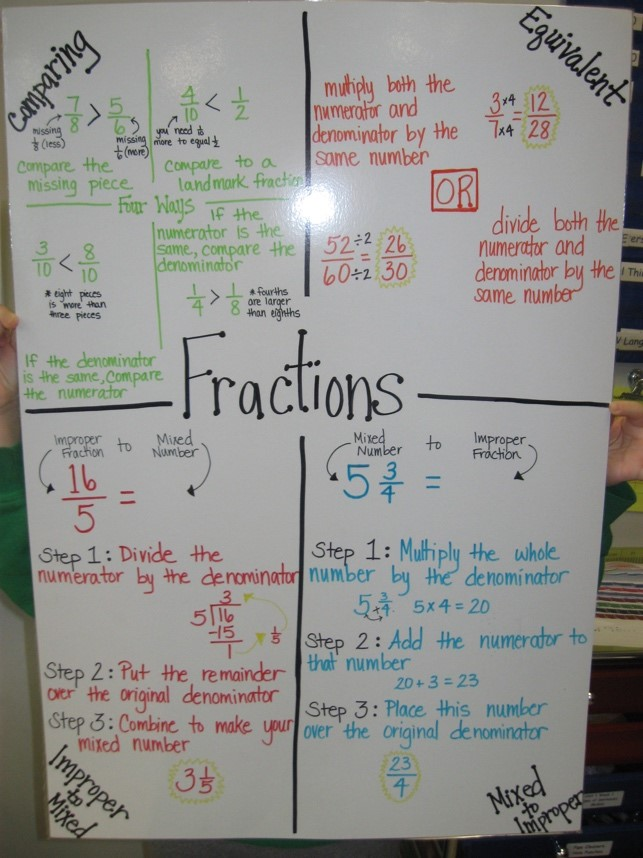### Number Sense:

Identify numbers, Count forward & backward # 1-10,000

Skip count- by 10s or 100s, forward and backward starting at different numbers.

Understand place value (ones, tens, hundreds, etc) and the value.

### Fractions:

#### Comparing Fractions

• Use Pictures, number lines, common denominators and common numerators to compare fractions to each other.
• Equivalent Fractions
• Multiple the numerator and denominator by the same number OR divide the numerator and denominator by the same number.

#### Improper Fraction to Mixed Number

• Step 1- Divide the numerator by the denominator.
• Step 2- Put the remainder over the original denominator.
• Step 3- Combine to make your mixed number.

#### Mixed Number to Improper Fraction

• Step 1- Multiple the whole number by the denominator.
• Step 2- Add the numerator to that number.
• Step 3- Place this number over the original denominator.Addition and subtraction facts within 10 should be automatic- if not, keep practicing! (5+3, 4+2, 8-1, 6-4)

#### Break Apart:

Break each number by place value.  Example: 238 = 200 + 30 + 8.  Line up decomposed number like a traditional subtraction equation.  Solve and regroup ones and tens as appropriate.

#### Picture/ Model:

represent each number using base 10 visuals.  square = hundreds, line = tens, dot = ones. Set up visual equation in tradition addition equation and solve, regrouping ones or tens as appropriate.

#### Place Value Chart:

Decompose each number into the correct column in the place value chart.  Solve and regroup ones and tens as appropriate.

#### Pull Down:

Represent the equation by place value, grouping the ones, tens, and hundreds together.  Solve and regroup ones and tens as appropriate.